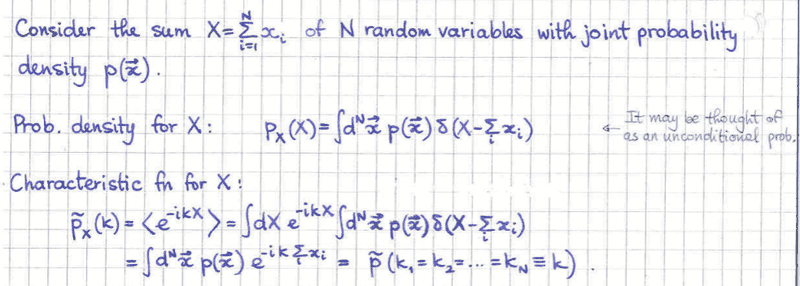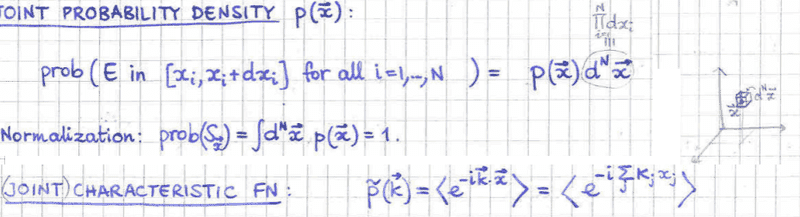# Characteristic function of the sum of random variables

## Homework StatementI am trying to understand the very last equality for (let me replace the tilda with a hat ) ##\hat{P_{X}(K)}=\hat{P(k_1=k_2=...=k_{N}=k)}##(1)

## Homework EquationsI also thought that the following imaginary exponential delta identity may be useful, due to the equality of the ##k_i##, but see comments below:

##\int dk \exp^{ikx} = \delta(x=0) ##

## The Attempt at a Solution

So it sees to me the goal is something like expressing ##\hat{P_{X}(K)}## in terms of ##P(\hat{k_i})## ?

So these are given by ##\hat{P(k_1)......P(k_n)}= \int d^{N} \vec{x} p(\vec{x}) \exp^{-i \sum\limits_j x_j k_j } ##

I thought Id first try to look at the simplified case of indepedent random variables to understand (1) but still cant seem to get it.

So in this case ##p(\vec{x}) = \Pi_{i} p(x_i)##

And then we have

(If I am correct in that the notation is that ##\Pi_{i} dx_i = d^N (\vec{x}) ##)
##\hat{P(k_1)......P(k_n)}= \int \Pi_{i} dx_i p(x_i) \exp^{-i \sum\limits_j x_j k_j } ##
and then you can seperate the integrals and so we have:

##\hat{P(k_1)......P(k_n)}= \hat{P_{x_1}(k_1)} ......\hat{P_{x_n}(k_n)} ## (2)

Now if I consider the independent case in ##\hat{P_{X}(K)}## I have:

##\hat{P_{X}(K)} =\int \Pi_{i} dx_i p(x_i) \exp^{-i k \sum\limits_j x_j }
= \int dx_1 p(x_1) e^{-ix_1 k} \int dx_2 p(x_2) e^{-ix_2 k}...\int dx_N p(x_N) e^{-ikx_N}
= \hat{P_{x_1}(k)} ......\hat{P_{x_n}(k)} ##

So if I compare this to (2), and can reason( I'm not sure you can) that it does matter whether you have ##k_i## or ##k##, this is just the label of the fourier transform, but look at the lower notation that gives the distribution, that ##\hat{P_{x_1}(k)}= \hat{P_{x_1}(k_1)} ## and then I have ##k_1=k## and can do the same for each ##k_i## etc.

##\int d^{N} \vec{x} p(\vec{x}) \exp^{-i k \sum\limits_j x_j } ##

and I can't see how you can make any conclusions without knowing what ## p(\vec{x}) ## is?

Many thanks

tnich
Homework Helper

## Homework Statement

View attachment 226899

I am trying to understand the very last equality for (let me replace the tilda with a hat ) ##\hat{P_{X}(K)}=\hat{P(k_1=k_2=...=k_{N}=k)}##(1)

## Homework Equations

View attachment 226898

I also thought that the following imaginary exponential delta identity may be useful, due to the equality of the ##k_i##, but see comments below:

##\int dk \exp^{ikx} = \delta(x=0) ##

## The Attempt at a Solution

So it sees to me the goal is something like expressing ##\hat{P_{X}(K)}## in terms of ##P(\hat{k_i})## ?

So these are given by ##\hat{P(k_1)......P(k_n)}= \int d^{N} \vec{x} p(\vec{x}) \exp^{-i \sum\limits_j x_j k_j } ##

I thought Id first try to look at the simplified case of indepedent random variables to understand (1) but still cant seem to get it.

So in this case ##p(\vec{x}) = \Pi_{i} p(x_i)##

And then we have

(If I am correct in that the notation is that ##\Pi_{i} dx_i = d^N (\vec{x}) ##)
##\hat{P(k_1)......P(k_n)}= \int \Pi_{i} dx_i p(x_i) \exp^{-i \sum\limits_j x_j k_j } ##
and then you can seperate the integrals and so we have:

##\hat{P(k_1)......P(k_n)}= \hat{P_{x_1}(k_1)} ......\hat{P_{x_n}(k_n)} ## (2)

Now if I consider the independent case in ##\hat{P_{X}(K)}## I have:

##\hat{P_{X}(K)} =\int \Pi_{i} dx_i p(x_i) \exp^{-i k \sum\limits_j x_j }
= \int dx_1 p(x_1) e^{-ix_1 k} \int dx_2 p(x_2) e^{-ix_2 k}...\int dx_N p(x_N) e^{-ikx_N}
= \hat{P_{x_1}(k)} ......\hat{P_{x_n}(k)} ##

So if I compare this to (2), and can reason( I'm not sure you can) that it does matter whether you have ##k_i## or ##k##, this is just the label of the fourier transform, but look at the lower notation that gives the distribution, that ##\hat{P_{x_1}(k)}= \hat{P_{x_1}(k_1)} ## and then I have ##k_1=k## and can do the same for each ##k_i## etc.

##\int d^{N} \vec{x} p(\vec{x}) \exp^{-i k \sum\limits_j x_j } ##

and I can't see how you can make any conclusions without knowing what ## p(\vec{x}) ## is?

Many thanks
I don't see that you have considered convolution of the distribution functions here. The sum of two iid random variables ##X = X_1+X_2## has a pdf
##f(x) = \int_{-\infty}^{\infty}g(x-x_1)g(x_1)~dx_1##
where ##g(x_i)## is the pdf of the ##X_1## and ##X_2##.

tnich
Homework Helper
I don't see that you have considered convolution of the distribution functions here. The sum of two iid random variables ##X = X_1+X_2## has a pdf
##f(x) = \int_{-\infty}^{\infty}g(x-x_1)g(x_1)~dx_1##
where ##g(x_i)## is the pdf of the ##X_1## and ##X_2##.
Looking again at your problem statement, I can see that you are trying to do something with random variables that are not independent, but you never state the question that you want to answer. You say you can't draw any conclusions about ##p(\vec{x})##. What kind of conclusions do you want to draw?

Looking again at your problem statement, I can see that you are trying to do something with random variables that are not independent, but you never state the question that you want to answer. You say you can't draw any conclusions about ##p(\vec{x})##. What kind of conclusions do you want to draw?
see bottom of my OP under section 1. I am trying to understand the last equailty...?

tnich
Homework Helper
see bottom of my OP under section 1. I am trying to understand the last equailty...?
So am I. I am having trouble parsing the right-hand side of
##\tilde P_{X}(k)=\tilde P(k_1=k_2=...=k_{N}=k)##
It is a characteristic function, so it is the expectation of a function ##exp\{-ikf(x_1,x_2,. . .,x_n)\}##, and we know what that function looks like. But ##\tilde P(k_1=k_2=...=k_{N}=k)## seems to be a meaningless expression.

Ray Vickson
Homework Helper
Dearly Missed
So am I. I am having trouble parsing the right-hand side of
##\tilde P_{X}(k)=\tilde P(k_1=k_2=...=k_{N}=k)##
It is a characteristic function, so it is the expectation of a function ##exp\{-ikf(x_1,x_2,. . .,x_n)\}##, and we know what that function looks like. But ##\tilde P(k_1=k_2=...=k_{N}=k)## seems to be a meaningless expression.

If ##\tilde{P}_X(k_1, k_2, \ldots, k_n)= \tilde{P}_X(\mathbf{k})## is the (multivariate) characteristic function of the multivariate density ##p(x_1, x_2, \ldots, x_n) = p(\mathbf{x})##, then, by definition we have
$$\tilde{P}_X(\mathbf{k}) = \int \exp(-i \: \mathbf{k \cdot x})\, p(\mathbf{x}) \, d^n \mathbf{x}$$
Also, by definition, if ##f_Y(y)## is the density function of the random variable ##Y = X_1 + X_2 + \cdots + X_n##, its characteristic function is
$$\tilde{P}_Y(k) = \int \exp(-i \, k y) f_Y(y) \, dy.$$
However, because of the Law of the Unconcious Statistician (LOTUS), we can write
$$\tilde{P}_Y(k) = \int \exp(-i k \sum_j x_j)\, p(\mathbf{x}) \, d^n \mathbf{x} \hspace{3em} (1)$$
(Note that in principle, we cannot simply write this down; we need to justify it. That is where the LOTUS comes in.)

Once we have equation (1) we can simply say that
$$\tilde{P}_Y(k) = \tilde{P}_X(k,k,k,\ldots,k).$$
That is probably what the author meant by his/her ill-advised notation.

For more on the LOTUS, see, eg.,
https://en.wikipedia.org/wiki/Law_of_the_unconscious_statistician (just the bare basics, with no proofs)
or
https://math.stackexchange.com/questions/415196/proving-the-law-of-the-unconscious-statistician (statement and proof)

Apparently, some authors have dropped that name in newer additions of their books, because of complaints from statisticians. Nevertheless, some other books have shown the continuing need for such a result, because some authors really do use the result 'unconsciously", or at least, without alerting the reader to the issue.

Last edited:
•tnich
tnich
Homework Helper
If ##\tilde{P}_X(k_1, k_2, \ldots, k_n)= \tilde{P}_X(\mathbf{k})## is the (multivariate) characteristic function of the multivariate density ##p(x_1, x_2, \ldots, x_n) = p(\mathbf{x})##, then, by definition we have
$$\tilde{P}_X(\mathbf{k}) = \int \exp(-i \: \mathbf{k \cdot x})\, p(\mathbf{x}) \, d^n \mathbf{x}$$
Also, by definition, if ##f_Y(y)## is the density function of the random variable ##Y = X_1 + X_2 + \cdots + X_n##, its characteristic function is
$$\tilde{P}_Y(k) = \int \exp(-i \, k y) f_Y(y) \, dy.$$
However, because of the Law of the Unconcious Statistician (LOTUS), we can write
$$\tilde{P}_Y(k) = \int \exp(-i k \sum_j x_j)\, p(\mathbf{x}) \, d^n \mathbf{x} \hspace{3em} (1)$$
(Note that in principle, we cannot simply write this down; we need to justify it. That is where the LOTUS comes in.)

Once we have equation (1) we can simply say that
$$\tilde{P}_Y(k) = \tilde{P}_X(k,k,k,\ldots,k).$$
That is probably what the author meant by his/her ill-advised notation.

For more on the LOTUS, see, eg.,
https://en.wikipedia.org/wiki/Law_of_the_unconscious_statistician (just the bare basics, with no proofs)
or
https://math.stackexchange.com/questions/415196/proving-the-law-of-the-unconscious-statistician (statement and proof)

Apparently, some authors have dropped that name in newer additions of their books, because of complaints from statisticians. Nevertheless, some other books have shown the continuing need for such a result, because some authors really do use the result 'unconsciously", or at least, without alerting the reader to the issue.
Thanks! I think you meant ##\tilde{P}_Y(k) = \tilde{P}_X(k,k,k,\ldots,k)##, and you have given a good answer to the question in the OP about what that equality means.